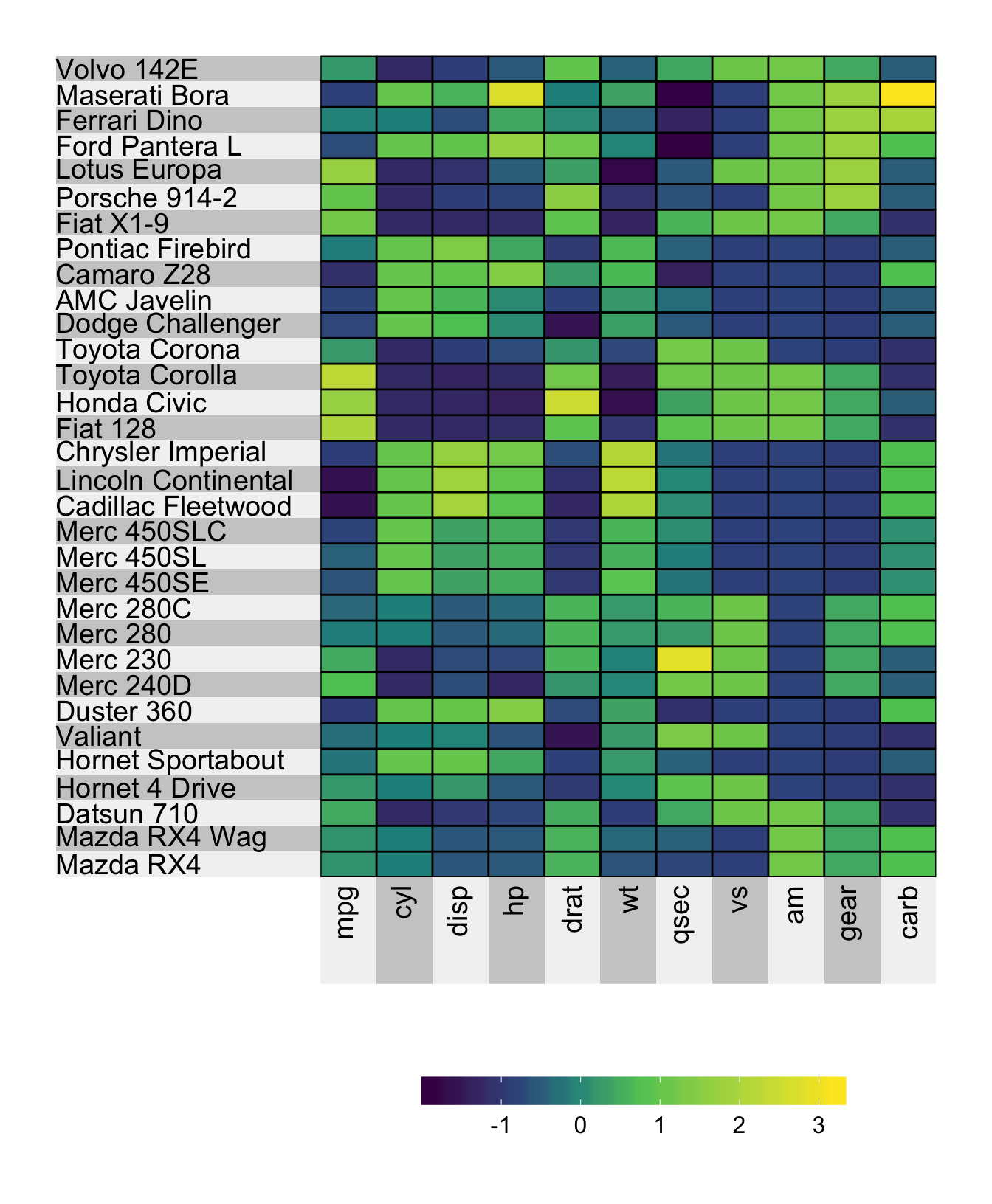# Chapter 10 Labels

The default labels alternate between light and dark grey, and disappear when you have more than 100 rows or columns. In this situation, to force the row or column labels to appear, you can set force.left.label = TRUE/force.bottom.label = TRUE.

## 10.1 Removing the labels

To remove either the row or column labels, you can use the left.label and bottom.label arguments.

superheat(X = mtcars, # heatmap matrix
# remove the labels
left.label = "none",
bottom.label = "none",
# scale the matrix columns
scale = TRUE)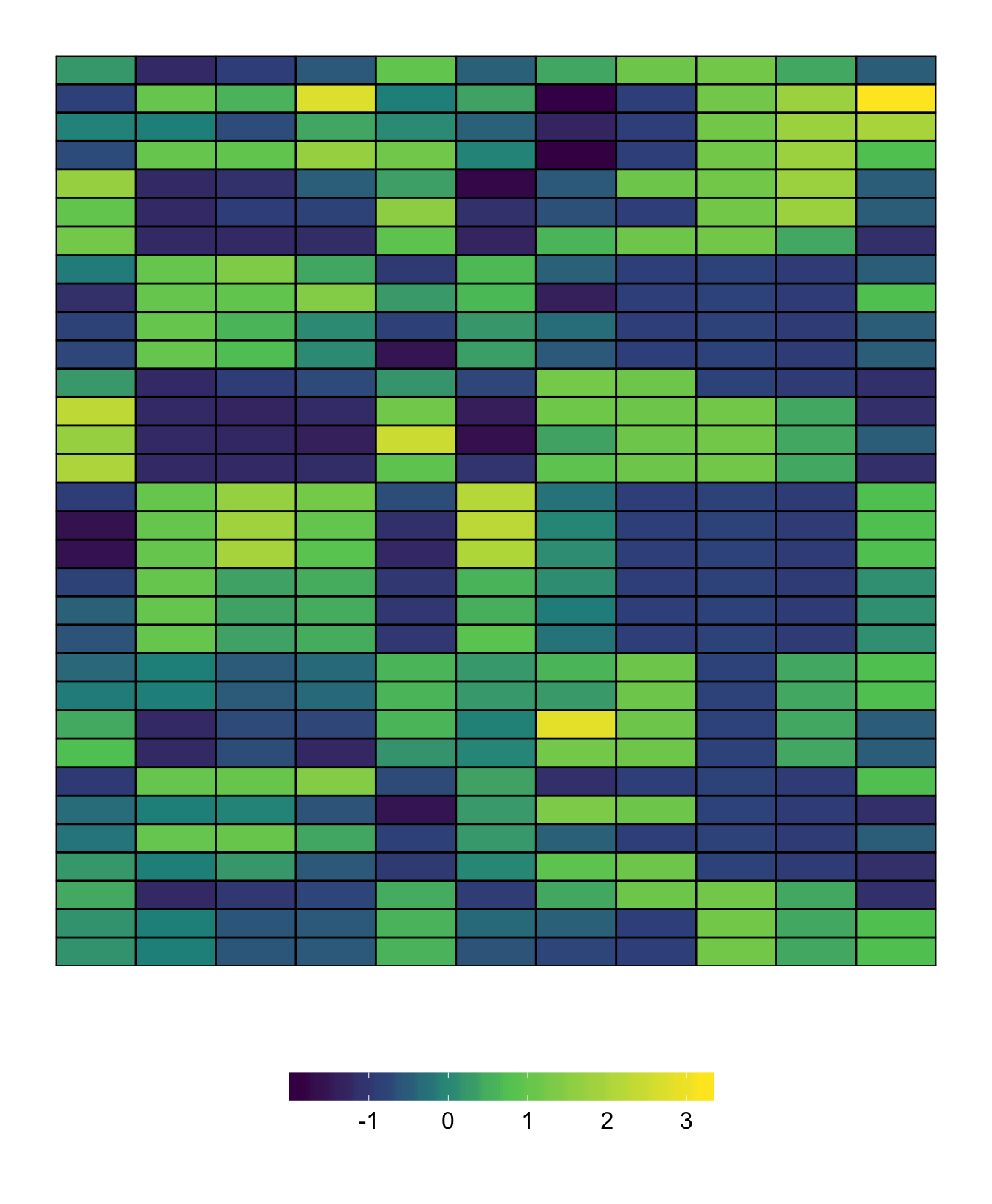Recall that if you have clustered your matrix and you want the labels to show the variable names, you can set left.label = "variable" and bottom.label = "variable"

## 10.2 Label size

Although the label sizes are designed to be a little bit “smart”, the size of the labels can be changed using left.label.size and bottom.label.size. Note that this changes the size of the label bar but does not change the text size.

superheat(X = mtcars, # heatmap matrix
# change the size of the labels
left.label.size = 0.4,
bottom.label.size = 0.1,
# scale the matrix columns
scale = TRUE)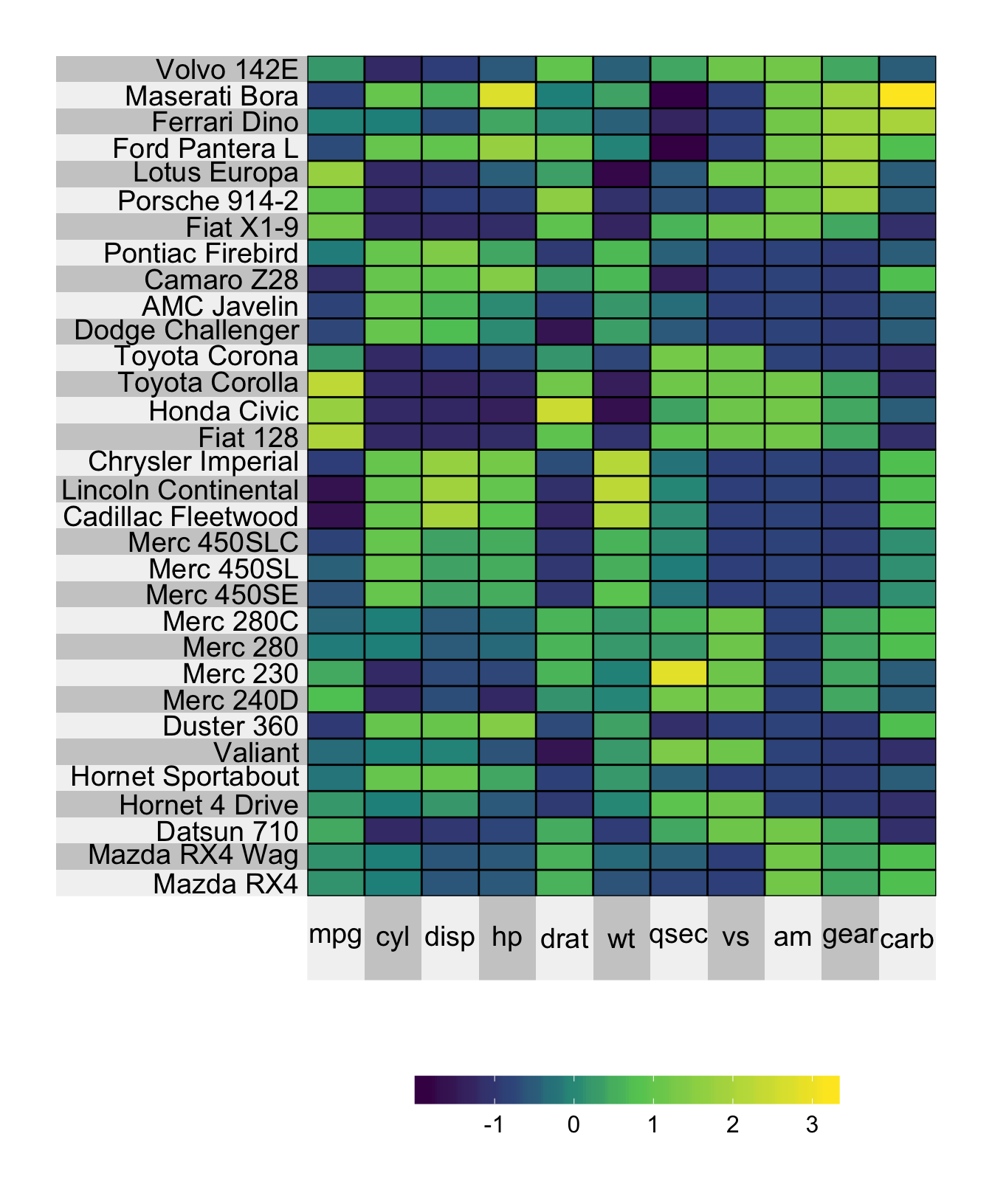## 10.3 Label color

Changing the color of the labels can be done using the left.label.col and bottom.label.col arguments. You can provide a single color or you can provide a vector of colors (in which case this vector will be cycled through to fill the length of the labels.)

superheat(X = mtcars, # heatmap matrix
# change the color of the labels
left.label.col = "white",
bottom.label.col = c("#b3e2cd","#fdcdac","#e5d8bd"),
# scale the matrix columns
scale = TRUE)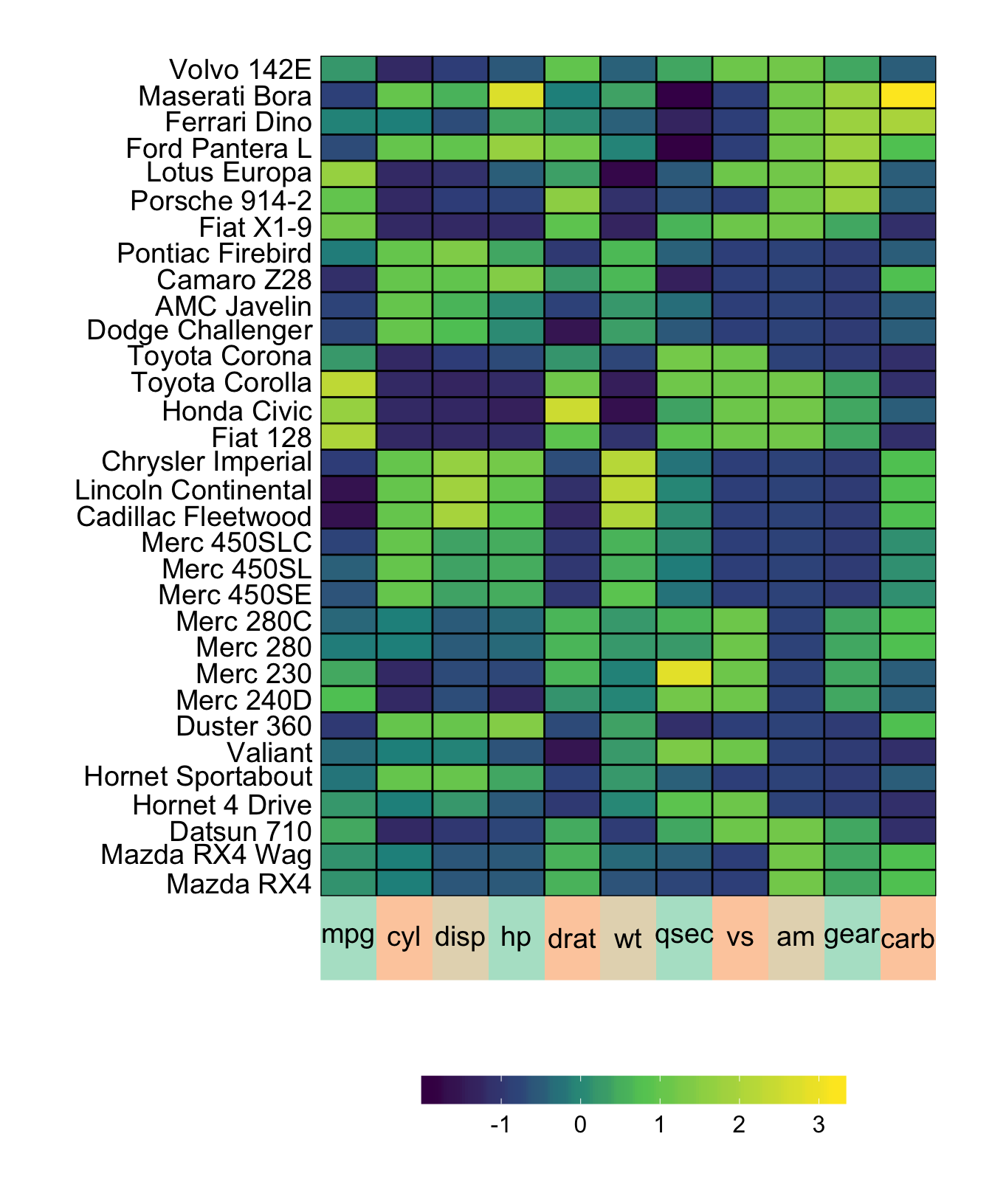## 10.4 Text size

The size of the label text can be changed using the left.label.text.size and bottom.label.text.size arguments.

superheat(X = mtcars, # heatmap matrix
# change the size of the label text
left.label.text.size = 8,
bottom.label.text.size = 6,
# scale the matrix columns
scale = TRUE)## 10.5 Text color

Changing the color of the text can be achieved using the left.label.text.col and bottom.label.text.col arguments.

superheat(X = mtcars, # heatmap matrix
# change the color of the label text
left.label.text.col = "red",
bottom.label.text.col = c("orange","cornflowerblue"),
# scale the matrix columns
scale = TRUE)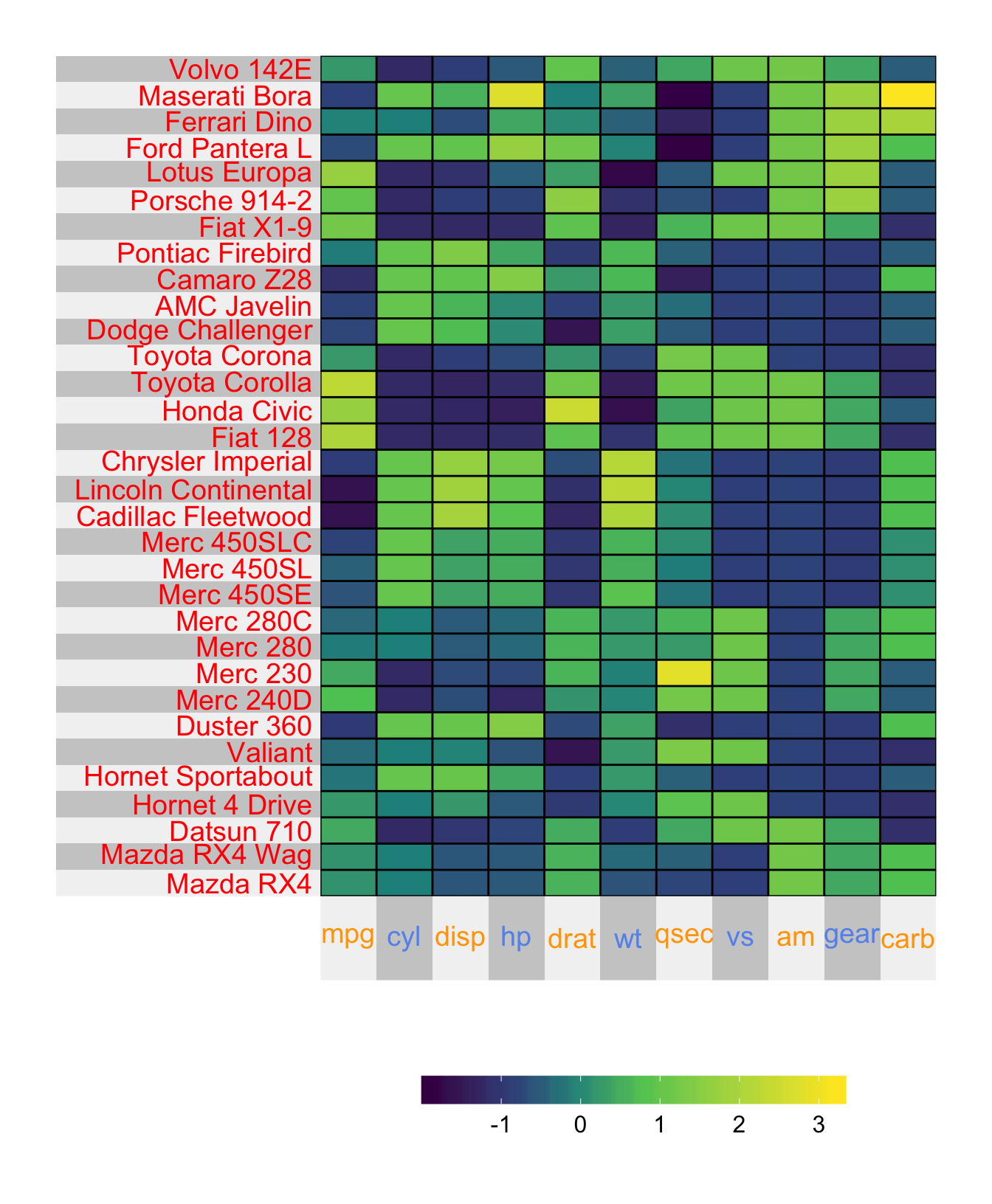## 10.6 Text angle

By default, the text has angle 0. Rotating the text by $$x$$ degrees can be achieved using left.label.text.angle = x or bottom.label.text.angle = x.

superheat(X = mtcars, # heatmap matrix
# change the angle of the label text
bottom.label.text.angle = 90,
# scale the matrix columns
scale = TRUE)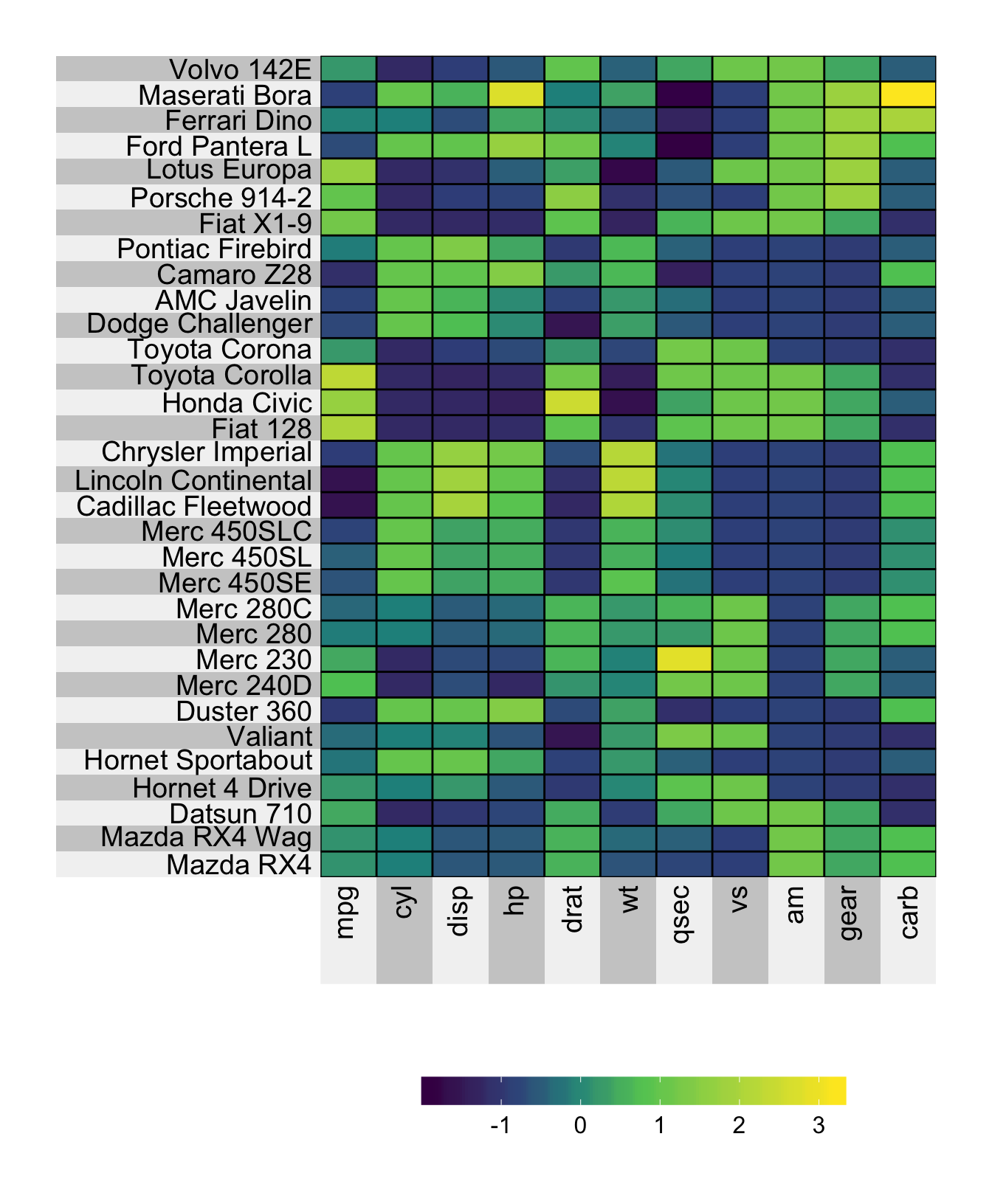## 10.7 Text alignment

By default, the text is center-aligned. Changing to right-aligned or left-aligned can be achieved using the left.label.text.alignment and bottom.label.text.alignment arguments.

superheat(X = mtcars, # heatmap matrix
# change the angle of the label text
bottom.label.text.angle = 90,
left.label.text.alignment = "left",
bottom.label.text.alignment = "right",
# scale the matrix columns
scale = TRUE)